The OpenFOAM Foundation
BarycentricTensor< Cmpt > Class Template Reference

Templated 4x3 tensor derived from VectorSpace. Has 12 components. Can represent a barycentric transformation as a matrix-barycentric inner- product. Can alternatively represent an inverse barycentric transformation as a vector-matrix inner-product. More...

Inheritance diagram for BarycentricTensor< Cmpt >: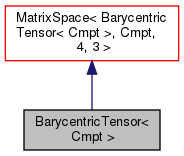[legend]
Collaboration diagram for BarycentricTensor< Cmpt >:[legend]

## Public Types

enum  components {
XA, XB, XC, XD,
YA, YB, YC, YD,
ZA, ZB, ZC, ZD
}
Component labeling enumeration. More...

typedef Tensor< labellabelType
Equivalent type of labels used for valid component indexing. More...Public Types inherited from MatrixSpace< BarycentricTensor< Cmpt >, Cmpt, 4, 3 >
typedef MatrixSpace< BarycentricTensor< Cmpt >, Cmpt, Mrows, Ncols > msType
MatrixSpace type. More...Public Types inherited from VectorSpace< BarycentricTensor< Cmpt >, Cmpt, Mrows *Ncols >
typedef VectorSpace< BarycentricTensor< Cmpt >, Cmpt, Ncmpts > vsType
VectorSpace type. More...

typedef Cmpt cmptType
Component type. More...

## Public Member Functions

BarycentricTensor ()
Construct null. More...

BarycentricTensor (const Foam::zero)
Construct initialised to zero. More...

BarycentricTensor (const Barycentric< Cmpt > &x, const Barycentric< Cmpt > &y, const Barycentric< Cmpt > &z)
Construct given three barycentric components (rows) More...

BarycentricTensor (const Vector< Cmpt > &a, const Vector< Cmpt > &b, const Vector< Cmpt > &c, const Vector< Cmpt > &d)
Construct given four vector components (columns) More...

Barycentric< Cmpt > x () const

Barycentric< Cmpt > y () const

Barycentric< Cmpt > z () const

Vector< Cmpt > a () const

Vector< Cmpt > b () const

Vector< Cmpt > c () const

Vector< Cmpt > d () constPublic Member Functions inherited from MatrixSpace< BarycentricTensor< Cmpt >, Cmpt, 4, 3 >
MatrixSpace ()
Construct null. More...

MatrixSpace (const Foam::zero)
Construct initialized to zero. More...

MatrixSpace (const VectorSpace< Form2, Cmpt2, Mrows *Ncols > &)
Construct as copy of a VectorSpace with the same size. More...

MatrixSpace (const Block2< BarycentricTensor< Cmpt >, BRowStart, BColStart > &block)
Construct from a block of another matrix space. More...

MatrixSpace (Istream &)
Construct from Istream. More...

const Cmpt & elmt () const
Fast const element access using compile-time addressing. More...

Cmpt & elmt ()
Fast element access using compile-time addressing. More...

const Cmpt & xx () const

Cmpt & xx ()

const Cmpt & xy () const

Cmpt & xy ()

const Cmpt & xz () const

Cmpt & xz ()

const Cmpt & yx () const

Cmpt & yx ()

const Cmpt & yy () const

Cmpt & yy ()

const Cmpt & yz () const

Cmpt & yz ()

const Cmpt & zx () const

Cmpt & zx ()

const Cmpt & zy () const

Cmpt & zy ()

const Cmpt & zz () const

Cmpt & zz ()

typeOfTranspose< Cmpt, BarycentricTensor< Cmpt > >::type T () const
Return the transpose of the matrix. More...

ConstBlock< SubTensor, BRowStart, BColStart > block () const
Return a const sub-block corresponding to the specified type. More...

Block< SubTensor, BRowStart, BColStart > block ()
Return a sub-block corresponding to the specified type. More...

Foam::MatrixSpace< BarycentricTensor< Cmpt >, Cmpt, Mrows, Ncols >::template ConstBlock< SubTensor, BRowStart, BColStart > block () const

Foam::MatrixSpace< BarycentricTensor< Cmpt >, Cmpt, Mrows, Ncols >::template Block< SubTensor, BRowStart, BColStart > block ()

const Cmpt & operator() (const direction &i, const direction &j) const
(i, j) const element access operator More...

Cmpt & operator() (const direction &i, const direction &j)
(i, j) element access operator More...

void operator= (const Foam::zero)
Assignment to zero. More...

void operator= (const Block2< BarycentricTensor< Cmpt >, BRowStart, BColStart > &block)
Assignment to a block of another matrix space. More...

void operator&= (const MatrixSpace< BarycentricTensor< Cmpt >, Cmpt, Ncols, Ncols > &matrix)
Inner product with a compatible square matrix. More...Public Member Functions inherited from VectorSpace< BarycentricTensor< Cmpt >, Cmpt, Mrows *Ncols >
VectorSpace ()
Construct null. More...

VectorSpace (const Foam::zero)
Construct initialized to zero. More...

VectorSpace (Istream &)
Construct from Istream. More...

VectorSpace (const VectorSpace< Form2, Cmpt2, Ncmpts > &)
Construct as copy of a VectorSpace with the same size. More...

const Cmpt & component (const direction) const

Cmpt & component (const direction)

void component (Cmpt &, const direction) const

void replace (const direction, const Cmpt &)

const ConstBlock< SubVector, BStart > block () const

const VectorSpace< BarycentricTensor< Cmpt >, Cmpt, Ncmpts >::template ConstBlock< SubVector, BStart > block () const

const Cmpt & operator[] (const direction) const

Cmpt & operator[] (const direction)

void operator+= (const VectorSpace< BarycentricTensor< Cmpt >, Cmpt, Ncmpts > &)

void operator-= (const VectorSpace< BarycentricTensor< Cmpt >, Cmpt, Ncmpts > &)

void operator= (const Foam::zero)

void operator*= (const scalar)

void operator/= (const scalar)

## Static Public Attributes

static const direction rank = 2
Rank of BarycentricTensor is 2. More...Static Public Attributes inherited from MatrixSpace< BarycentricTensor< Cmpt >, Cmpt, 4, 3 >
static const direction mRows

static const direction nColsStatic Public Attributes inherited from VectorSpace< BarycentricTensor< Cmpt >, Cmpt, Mrows *Ncols >
static const direction dim
Dimensionality of space. More...

static const direction nComponents
Number of components in this vector space. More...

static const direction mRows

static const direction nCols

static const char *const typeName

static const char *const componentNames []

static const BarycentricTensor< Cmpt > zero

static const BarycentricTensor< Cmpt > one

static const BarycentricTensor< Cmpt > max

static const BarycentricTensor< Cmpt > min

static const BarycentricTensor< Cmpt > rootMax

static const BarycentricTensor< Cmpt > rootMinStatic Public Member Functions inherited from MatrixSpace< BarycentricTensor< Cmpt >, Cmpt, 4, 3 >
static direction m ()
Return the number of rows. More...

static direction n ()
Return the number of columns. More...

static msType identity ()
Return the identity matrix for square matrix spaces. More...Static Public Member Functions inherited from VectorSpace< BarycentricTensor< Cmpt >, Cmpt, Mrows *Ncols >
static direction size ()
Return the number of elements in the VectorSpace = Ncmpts. More...

static BarycentricTensor< Cmpt > uniform (const Cmpt &s)
Return a VectorSpace with all elements = s. More...Public Attributes inherited from VectorSpace< BarycentricTensor< Cmpt >, Cmpt, Mrows *Ncols >
Cmpt v_ [Ncmpts]
The components of this vector space. More...

## Detailed Description

### template<class Cmpt> class Foam::BarycentricTensor< Cmpt >

Templated 4x3 tensor derived from VectorSpace. Has 12 components. Can represent a barycentric transformation as a matrix-barycentric inner- product. Can alternatively represent an inverse barycentric transformation as a vector-matrix inner-product.

Source files

Definition at line 54 of file BarycentricTensor.H.

## ◆ labelType

 typedef Tensor

Equivalent type of labels used for valid component indexing.

Definition at line 61 of file BarycentricTensor.H.

## ◆ components

 enum components

Component labeling enumeration.

Enumerator
XA
XB
XC
XD
YA
YB
YC
YD
ZA
ZB
ZC
ZD

Definition at line 71 of file BarycentricTensor.H.

## ◆ BarycentricTensor() [1/4]

 BarycentricTensor ( )
inline

Construct null.

Definition at line 29 of file BarycentricTensorI.H.

Referenced by BarycentricTensor< Cmpt >::BarycentricTensor().

Here is the caller graph for this function: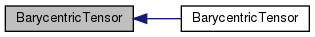## ◆ BarycentricTensor() [2/4]

 BarycentricTensor ( const Foam::zero )
inline

Construct initialised to zero.

Definition at line 34 of file BarycentricTensorI.H.

Here is the call graph for this function: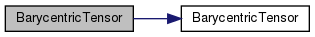## ◆ BarycentricTensor() [3/4]

 BarycentricTensor ( const Barycentric< Cmpt > & x, const Barycentric< Cmpt > & y, const Barycentric< Cmpt > & z )
inline

Construct given three barycentric components (rows)

Definition at line 42 of file BarycentricTensorI.H.

Here is the call graph for this function: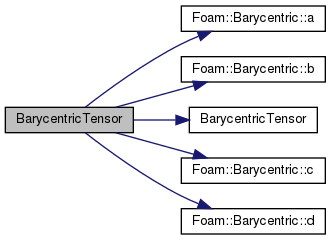## ◆ BarycentricTensor() [4/4]

 BarycentricTensor ( const Vector< Cmpt > & a, const Vector< Cmpt > & b, const Vector< Cmpt > & c, const Vector< Cmpt > & d )
inline

Construct given four vector components (columns)

Definition at line 67 of file BarycentricTensorI.H.

Here is the call graph for this function: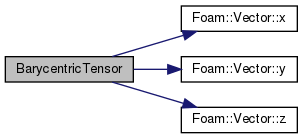## ◆ x()

 Foam::Barycentric< Cmpt > x ( ) const
inline

Definition at line 94 of file BarycentricTensorI.H.

Referenced by Foam::operator &().

Here is the caller graph for this function: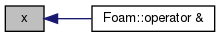## ◆ y()

 Foam::Barycentric< Cmpt > y ( ) const
inline

Definition at line 108 of file BarycentricTensorI.H.

Referenced by Foam::operator &().

Here is the caller graph for this function: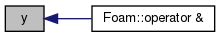## ◆ z()

 Foam::Barycentric< Cmpt > z ( ) const
inline

Definition at line 122 of file BarycentricTensorI.H.

Referenced by Foam::operator &().

Here is the caller graph for this function: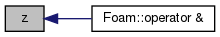## ◆ a()

 Foam::Vector< Cmpt > a ( ) const
inline

Definition at line 136 of file BarycentricTensorI.H.

Referenced by Foam::operator &().

Here is the caller graph for this function: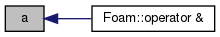## ◆ b()

 Foam::Vector< Cmpt > b ( ) const
inline

Definition at line 143 of file BarycentricTensorI.H.

Referenced by Foam::operator &().

Here is the caller graph for this function: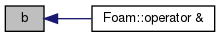## ◆ c()

 Foam::Vector< Cmpt > c ( ) const
inline

Definition at line 150 of file BarycentricTensorI.H.

Referenced by Foam::operator &().

Here is the caller graph for this function: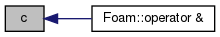## ◆ d()

 Foam::Vector< Cmpt > d ( ) const
inline

Definition at line 157 of file BarycentricTensorI.H.

Referenced by Foam::operator &().

Here is the caller graph for this function: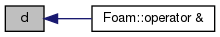## ◆ rank

 const direction rank = 2
static

Rank of BarycentricTensor is 2.

Definition at line 67 of file BarycentricTensor.H.

The documentation for this class was generated from the following files: